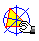CIRCUMCEVIAN TRIANGLE

The circumcevian triangle of the point P with respect to the triangle ABC is the triangle having as vertices the intersections of the cevians AP, BP and CP with the circumcircle. Two particular cases are: the circumcevian triangle of the centroid is the circummedial triangle.Circumcevian_triangle.mac